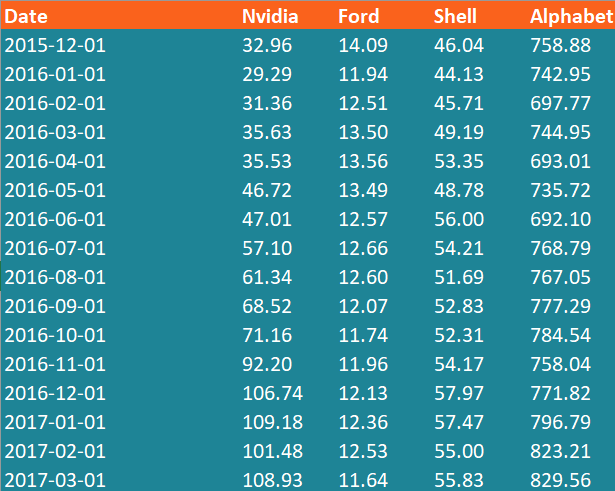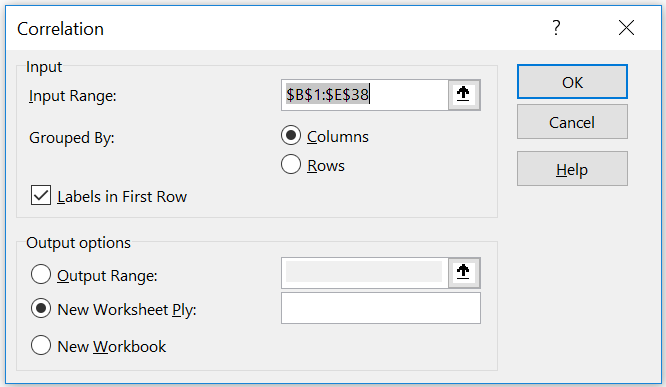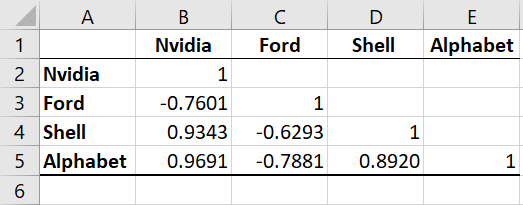# Correlation Matrix

A table that captures the correlation coefficients between different variables

## What is a Correlation Matrix?

A correlation matrix is simply a table which displays the correlation coefficients for different variables. The matrix depicts the correlation between all the possible pairs of values in a table. It is a powerful tool to summarize a large dataset and to identify and visualize patterns in the given data.

A correlation matrix consists of rows and columns that show the variables. Each cell in a table contains the correlation coefficient.

In addition, the correlation matrix is frequently utilized in conjunction with other types of statistical analysis. For instance, it may be helpful in the analysis of multiple linear regression models. Remember that the models contain several independent variables. In multiple linear regression, the correlation matrix determines the correlation coefficients between the independent variables in a model.

### How to Create a Correlation Matrix in Excel?

In order to understand the necessary steps in creating a correlation matrix in Excel, let’s consider the following example. You are the stock analyst in the investment bank. Your manager recently asked you to analyze the correlations between prices of stocks that can be potentially added to the portfolio. You then analyze the stocks of the following companies: NVIDIA, Ford, Shell, and Alphabet.

The best way to analyze the correlations between the stock prices of the abovementioned companies is to create a correlation matrix. It can be done through the following steps:

1. Download the data into Excel and arrange the data into the columns.

Each column represents the stock prices of a distinct company for the specified period (from December 2015 to November 2018).1. Click Data -> Data Analysis -> Correlation
2. Enter the input range that contains the name of the companies and the stock prices.
3. Ensure that Grouped By: Columns option is chosen (because our data is arranged in the columns).
4. Ensure that Labels in First Row option is chosen (the first rows of each column contain the names of the companies).
5. Choose the desired output option (i.e., the location on the spreadsheet where the correlation matrix will appear).
6. Click OK.Your matrix should look like the image below:CFI offers the Financial Modeling & Valuation Analyst (FMVA)™ certification program for those looking to take their careers to the next level. To keep learning and advancing your career, the following CFI resources will be helpful:

• Covariance
• Hypothesis Testing
• PEARSON Function
• Poisson Distribution

### Free Excel Tutorial

To master the art of Excel, check out CFI’s FREE Excel Crash Course, which teaches you how to become an Excel power user.  Learn the most important formulas, functions, and shortcuts to become confident in your financial analysis.

Launch CFI’s Free Excel Course now to take your career to the next level and move up the ladder!